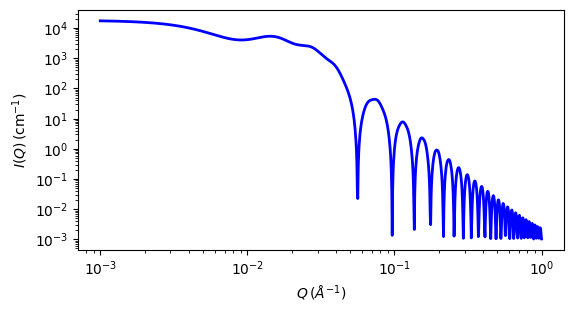# pearl_necklace

Colloidal spheres chained together with no preferential orientation

Parameter

Description

Units

Default value

scale

Scale factor or Volume fraction

None

1

background

Source background

cm-1

0.001

Mean radius of the chained spheres

80

edge_sep

Mean separation of chained particles

350

thick_string

2.5

num_pearls

Number of pearls in the necklace (must be integer)

none

3

sld

Scattering length density of the chained spheres

10-6-2

1

sld_string

Scattering length density of the chain linkage

10-6-2

1

sld_solvent

Scattering length density of the solvent

10-6-2

6.3

The returned value is scaled to units of cm-1 sr-1, absolute scale.

This model provides the form factor for a pearl necklace composed of two elements: N pearls (homogeneous spheres of radius R) freely jointed by M rods (like strings - with a total mass Mw = M * mr + N * ms, and the string segment length (or edge separation) l (= A - 2R)). A is the center-to-center pearl separation distance.

Definition

The output of the scattering intensity function for the pearl_necklace is given by (Schweins, 2004)

$I(q)=\frac{ \text{scale} }{V} \cdot \frac{(S_{ss}(q)+S_{ff}(q)+S_{fs}(q))} {(M \cdot m_f + N \cdot m_s)^2} + \text{bkg}$

where

$\begin{split}S_{ss}(q) &= 2m_s^2\psi^2(q)\left[\frac{N}{1-sin(qA)/qA}-\frac{N}{2}- \frac{1-(sin(qA)/qA)^N}{(1-sin(qA)/qA)^2}\cdot\frac{sin(qA)}{qA}\right] \\ S_{ff}(q) &= m_r^2\left[M\left\{2\Lambda(q)-\left(\frac{sin(ql/2)}{ql/2}\right)\right\}+ \frac{2M\beta^2(q)}{1-sin(qA)/qA}-2\beta^2(q)\cdot \frac{1-(sin(qA)/qA)^M}{(1-sin(qA)/qA)^2}\right] \\ S_{fs}(q) &= m_r \beta (q) \cdot m_s \psi (q) \cdot 4\left[ \frac{N-1}{1-sin(qA)/qA}-\frac{1-(sin(qA)/qA)^{N-1}}{(1-sin(qA)/qA)^2} \cdot \frac{sin(qA)}{qA}\right] \\ \psi(q) &= 3 \cdot \frac{sin(qR)-(qR)\cdot cos(qR)}{(qR)^3} \\ \Lambda(q) &= \frac{\int_0^{ql}\frac{sin(t)}{t}dt}{ql} \\ \beta(q) &= \frac{\int_{qR}^{q(A-R)}\frac{sin(t)}{t}dt}{ql}\end{split}$

where the mass mi is (SLDi - SLDsolvent) * (volume of the N pearls/rods). V is the total volume of the necklace.

Note

num_pearls must be an integer.

The 2D scattering intensity is the same as $$P(q)$$ above, regardless of the orientation of the q vector.Fig. 33 1D plot corresponding to the default parameters of the model.

Source

pearl_necklace.py $$\ \star\$$ pearl_necklace.c $$\ \star\$$ sas_3j1x_x.c $$\ \star\$$ sas_Si.c

References

1. R Schweins and K Huber, Particle Scattering Factor of Pearl Necklace Chains, Macromol. Symp. 211 (2004) 25-42 2004

1. Onsager, Ann. New York Acad. Sci., 51 (1949) 627-659

Authorship and Verification

• Author: